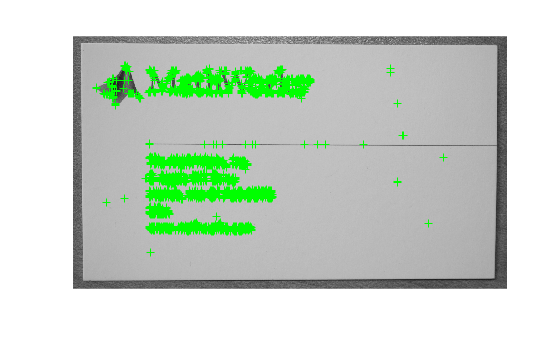# detectORBFeatures

Detect ORB keypoints and return an `ORBPoints` object

## Syntax

``points = detectORBFeatures(I)``
``points = detectORBFeatures(I,Name,Value)``

## Description

example

````points = detectORBFeatures(I)` returns an `ORBPoints` object that contains information about ORB keypoints. The ORB keypoints are detected from the input image by using the Oriented FAST and rotated BRIEF (ORB) feature detection method. ```

example

````points = detectORBFeatures(I,Name,Value)` specifies options using one or more name-value pair arguments.```

## Examples

collapse all

Read an image into the workspace.

`I = imread('businessCard.png');`

Convert the image into a grayscale image.

`I = im2gray(I);`

Display the grayscale image.

```figure imshow(I)```Detect and store ORB keypoints.

`points = detectORBFeatures(I);`

Display the grayscale image and plot the detected ORB keypoints. Suppress the display of circles around the detected keypoints. The ORB keypoints are detected in regions with high intensity variance.

```figure imshow(I) hold on plot(points,'ShowScale',false) hold off```Read a binary image into the workspace.

`I = imread('star.png');`

Display the image.

```figure imshow(I)```Detect and store ORB keypoints. Specify the scale factor for image decomposition as 1.01 and the number of decomposition levels as 3.

`points = detectORBFeatures(I,'ScaleFactor',1.01,'NumLevels',3);`

Display the image and plot the detected ORB keypoints. The inflection points in the binary shape image are detected as the ORB keypoints.

```figure imshow(I) hold on plot(points) hold off```## Input Arguments

collapse all

Input image, specified as an M-by-N grayscale image. The input image must be real and nonsparse.

Data Types: `single` | `double` | `int16` | `uint8` | `uint16` | `logical`

### Name-Value Arguments

Specify optional pairs of arguments as `Name1=Value1,...,NameN=ValueN`, where `Name` is the argument name and `Value` is the corresponding value. Name-value arguments must appear after other arguments, but the order of the pairs does not matter.

Before R2021a, use commas to separate each name and value, and enclose `Name` in quotes.

Example: `detectORBFeatures(I,'NumLevels',4)`

Scale factor for image decomposition, specified as the comma-separated pair consisting of `'ScaleFactor'` and a scalar greater than 1. The scale value at each level of decomposition is `ScaleFactor`(level-1), where level is any value in the range [0, `Numlevels`-1]. Given the input image of size M-by-N, the image size at each level of decomposition is $\frac{M}{ScaleFacto{r}^{\left(level\text{\hspace{0.17em}}-\text{\hspace{0.17em}}1\right)}}\text{\hspace{0.17em}}\text{\hspace{0.17em}}\text{-by-}\text{\hspace{0.17em}}\text{\hspace{0.17em}}\frac{N}{ScaleFacto{r}^{\left(level\text{\hspace{0.17em}}-\text{\hspace{0.17em}}1\right)}}$.

Data Types: `single` | `double` | `int8` | `int16` | `int32` | `int64` | `uint8` | `uint16` | `uint64` | `uint32`

Number of decomposition levels, specified as the comma-separated pair consisting of `'NumLevels'` and a scalar greater than or equal to 1. Increase this value to extract keypoints from the image at more levels of decomposition.

The number of decomposition levels for extracting keypoints is limited by the image size at that level. The image size at a level of decomposition must be at least 63-by-63 for detecting keypoints. The maximum level of decomposition is calculated as

If either the default value or the specified value of `'NumLevels'` is greater than levelmax, the function modifies `NumLevels` to levelmax and returns a warning.

Data Types: `single` | `double` | `int8` | `int16` | `int32` | `int64` | `uint8` | `uint16` | `uint64` | `uint32`

Region of interest for keypoint detection, specified as the comma-separated pair consisting of `'ROI'` and a vector of the format [x y width height]. The first two elements represent the location of the upper left corner of the region of interest. The last two elements represent the width and the height of the region of interest. The width and height of the region of interest must each be a value greater than or equal to 63.

## Output Arguments

collapse all

ORB keypoints, returned as an `ORBPoints` object. The object contains information about keypoints detected in the input image.

## Algorithms

The function detects keypoints from the input image by using the ORB feature detection method in .

 Rublee, E., V. Rabaud, K. Konolige, and G. Bradski. "ORB: An Efficient Alternative to SIFT or SURF." In Proceedings of the 2011 International Conference on Computer Vision, 2564–2571. Barcelona, Spain: IEEE, 2011.